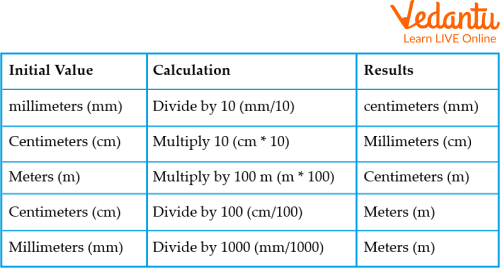Courses
Courses for Kids
Free study material
Free LIVE classes
More

# Introducing Cm to PercentageLIVE
Join Vedantu’s FREE Mastercalss

## Overview on Percentage

A percentage is a number or ratio that can be expressed as a fraction of 100. If we need to calculate percent of a number, divide the number by the whole and multiply by 100.

In academics, the marks obtained in any subject are calculated in terms of percentage. Ramesh got 78% of marks in his final exam. So, this percentage is calculated on account of the total marks obtained by Ramesh, in all subjects to the total marks.Percent means “per hundred”. 20% means 20 per 100. Percent always compares a quantity to 100 only.

A percent can be written as a fraction or a decimal.

Thus, 40% (as percentage) or $\dfrac{40}{100}$ (as fraction) or 0.40 (as decimal)

So many things in life involve percentage, from taxes to stock prices and more.

## Metric Measures as PercentagesMetrics conversion chartThe table is of important units to remember

The metric measures as percentages. Now we will learn how to convert the cent as percentage of a dollar, centimeter as percentage of a meter, and 1 cent is equal to how many meters.

Metric units of measurement are the metric units of measurements used for different quantities.

The metric system of units of measurement is based on powers of 10.

Prefixes are used to indicate which power of 10 is involved.

The prefix “centi” means $\dfrac{1}{100}$th

e.g. A centimeter is $\dfrac{1}{100}​$ th of a meter.

The prefix “milli” means $\dfrac{1}{1000}$ th

• Cm to percentage

Convert cm into percentage

1 % = 1 cm/m (cm per meter length).

Centimeter is a unit of length in the metric system, equal to one hundredth of a meter or 0.01  m.

For example, a slope of 100 % is equal to a slope of 100 cm in height for 1 m in length

• ### Cent as percentage of a dollar

100 cent = 1 dollar

1 cent = $\dfrac{1}{100}$ of a dollar

= 1 % of a dollar

So, we get, 2 cent

= 2 % of a dollar

10 cent = 10 % of a dollar

For example, to find the value of 4 % of $75 = \dfrac{4}{100} \times 75 = 3$

• ### Centimeter as percentage of a meter

100 cm = 1 meter

1 cm = $\dfrac{1}{100}$ of a meter

= 1 % of a meter

So, we get, 3 cm

= 3% of a meter

20 cm

= 20 % of a meter

For example, find the value of 5% of 120 m = $\dfrac{5}{100} × \times 120$ m = 6 m

• ### 1 cent is equal to how many square meters

Cent is a traditional unit of area measurement used majorly in India. This unit is widely used for measuring smaller plots and land parcels of around one acre.

These unit measures are used to measure the area of land.

40.46 square meter

One cent is equal to 40.46 square meters, and 1 square meter is 0.02471 cents.

## How to Calculate Cents?Conversion of cent to sq.feet

To convert cents to square feet, multiply the area in cents by 435.6.

Example: How much is 1 cent land?

Ans: 435.56 square feet

1 cent is equal to 435.56 square feet, and both are conventional land measurement units used to measure property size.

## Convert meter Square to Cent

To calculate square meter to cent, wherein square meter is equal to 0.02471 Cent, multiply the figure in square meter by 0.02471.

1 sq.mt = 0.02471 cents

Likewise, 2 sq.mt = 0.04942 cents

3 sq.mt = 0.07413 cents and so on.

## Solved Examples

Q1 Find a percentage?

20 % of 80=?

Ans: Percentage= rate x base

$\mathrm{P}=20 \% \times 80$

$\mathrm{P}=0.20 \times 80$

$\mathrm{P}=16$

Q2 Convert 356 centimeters to meters.

Ans: 1 cm=0.01 m

356 cm= 356 x 0.01

356 cm= 3.56 m

Therefore, 356 cm is equivalent to 3.56 m.

Q3 Convert 1000 cents into square meters.

Ans: We will multiply,

1000 x 40.468564224 = 40468.564224 square meters.

## Practice Questions

Q1 Rachel has a rope of length 40 m. She has given 12 m 53 cm to Sam, 18 m 35 cm to Ron and 9 m 7 cm to Jack. What length of rope is still left with Rachel?

Ans: 0.05 m

Q2 In an exam Ashley secured 332 marks. If she secured 83 % marks, find the maximum marks.

Ans: Ashley got 332 marks out of 400 marks.

Q3 3 Convert 75000 sq.cms to sq.mt

Ans: 7.5 square meters.

## Summary

In mathematics, a percentage is a way of expressing in numbers the proportional parts of something. For example, 70% is $\dfrac{7}{10}$. 0.7 in decimal form and 20% could be read as $\dfrac{2}{5}$ or 0.4 when written in decimal form. Metric measurements come from a long history of systems that used relative units as a form of measurement - like inches or pounds - that were later standardized into absolute units based on single units such as meters or kilogram.We studied about conversions of sq.m to cm sq., cent to sq.mt etc. These conversions make our everyday life easier because they are of much use.

Last updated date: 27th Sep 2023
Total views: 78.3k
Views today: 2.78k

## FAQs on Introducing Cm to Percentage

1. Which unit is commonly used in a metric system?

The three most common base units in the metric system are the meter, gram, and liter.

2. What is the metric based on?

The metric system is based upon powers of ten.

3. What are the three parts of percent?

The percent, the base (or whole), and the amount.